Angle

A straight line p given by the equation. Calculate the size of angle in degrees between line p and y-axis.

Result

x =  143.13 °

Solution:Leave us a comment of example and its solution (i.e. if it is still somewhat unclear...):Be the first to comment!To solve this example are needed these knowledge from mathematics:

For Basic calculations in analytic geometry is helpful line slope calculator. From coordinates of two points in the plane it calculate slope, normal and parametric line equation(s), slope, directional angle, direction vector, the length of segment, intersections the coordinate axes etc. Most natural application of trigonometry and trigonometric functions is a calculation of the triangles. Common and less common calculations of different types of triangles offers our triangle calculator. Word trigonometry comes from Greek and literally means triangle calculation.

Next similar examples:

1. Supplementary angles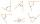One of the supplementary angles are three times larger than the other. What size is larger of supplementary angles?
2. Angle between lines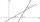Calculate the angle between these two lines: ? ?
3. The angle of lines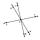Calculate the angle of two lines y=x-21 and y=-2x+14
4. SlopeFind the slope of the line: x=t and y=1+t.
5. SlopeWhat is the slope of a line with an inclination -221°?
6. Reference angleFind the reference angle of each angle: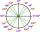What is greater angle? -0.7 radians or -0.7π radians?
8. Slope formFind the equation of a line given the point X(8, 1) and slope -2.8. Arrange your answer in the form y = ax + b, where a, b are the constants.
9. V - slopeThe slope of the line whose equation is -3x -9 = 0 is
10. Perpendicular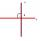Determine the slope of the line perpendicular to the line p: y = -x +4.
11. LineIt is true that the lines that do not intersect are parallel?
12. LineLine p passing through A[-10, 6] and has direction vector v=(3, 2). Is point B[7, 30] on the line p?
13. Two equationsSolve equations (use adding and subtracting of linear equations): -4x+11y=5 6x-11y=-5
14. TreesA certain species of tree grows an average of 0.5 cm per week. Write an equation for the sequence that represents the weekly height of this tree in centimeters if the measurements begin when the tree is 200 centimeters tall.
15. Theorem proveWe want to prove the sentence: If the natural number n is divisible by six, then n is divisible by three. From what assumption we started?
16. One halfOne half of ? is: ?
17. Functions f,gFind g(1) if g(x) = 3x - x2 Find f(5) if f(x) = x + 1/2